# 机器学习算法一览（1）群内定期组织分享

（2）确保群内分享者和学习者数量适合，有分享能力者不限名额，学习者数量少于分享者，按申请顺序排序。

2.机器学习算法简述

2.1 从机器学习问题角度分类

2.2 从算法的功能角度分类

2.2.1 回归算法(Regression Algorithms)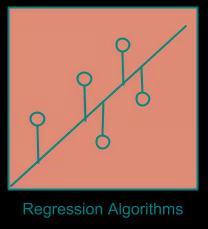Ordinary Least Squares Regression (OLSR)

Linear Regression

Logistic Regression

Stepwise Regression

Locally Estimated Scatterplot Smoothing (LOESS)

Multivariate Adaptive Regression Splines (MARS)

2.2.2 基于实例的算法(Instance-based Algorithms)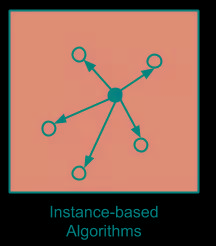k-Nearest Neighbour (kNN)

Learning Vector Quantization (LVQ)

Self-Organizing Map (SOM)

Locally Weighted Learning (LWL)

2.2.3 决策树类算法(Decision Tree Algorithms)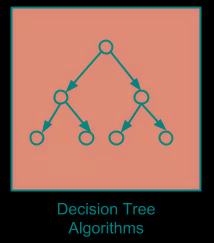Classification and Regression Tree (CART)

Iterative Dichotomiser 3 (ID3)

C4.5 and C5.0 (different versions of a powerful approach)

Chi-squared Automatic Interaction Detection (CHAID)

M5

Conditional Decision Trees

2.2.4 贝叶斯类算法(Bayesian Algorithms)

Naive Bayes

Gaussian Naive Bayes

Multinomial Naive Bayes

Averaged One-Dependence Estimators (AODE)

Bayesian Belief Network (BBN)

Bayesian Network (BN)

2.2.5 聚类算法(Clustering Algorithms)

k-Means

Hierarchical Clustering

Expectation Maximisation (EM)

2.2.6 关联规则算法(Association Rule Learning Algorithms)

Apriori algorithm

Eclat algorithm

2.2.7 人工神经网络类算法(Artificial Neural Network Algorithms)

Perceptron

Back-Propagation

Radial Basis Function Network (RBFN)

2.2.8 深度学习(Deep Learning Algorithms)

Deep Boltzmann Machine (DBM)

Deep Belief Networks (DBN)

Convolutional Neural Network (CNN)

Stacked Auto-Encoders

2.2.9 降维算法(Dimensionality Reduction Algorithms)

Principal Component Analysis (PCA)

Principal Component Regression (PCR)

Partial Least Squares Regression (PLSR)

Sammon Mapping

Multidimensional Scaling (MDS)

Linear Discriminant Analysis (LDA)

Mixture Discriminant Analysis (MDA)

Quadratic Discriminant Analysis (QDA)

Flexible Discriminant Analysis (FDA)

2.2.10 模型融合算法(Ensemble Algorithms)

Random Forest

Boosting

Bootstrapped Aggregation (Bagging)

Stacked Generalization (blending)

Gradient Boosting Machines (GBM)

Gradient Boosted Regression Trees (GBRT)

2.3 机器学习算法使用图谱

scikit-learn作为一个丰富的python机器学习库，实现了绝大多数机器学习的算法，有相当多的人在使用，于是我这里很无耻地把machine learning cheat sheet for sklearn搬过来了，原文可以看这里。哈哈，既然讲机器学习，我们就用机器学习的语言来解释一下，这是针对实际应用场景的各种条件限制，对scikit-learn里完成的算法构建的一颗决策树，每一组条件都是对应一条路径，能找到相对较为合适的一些解决方法，具体如下：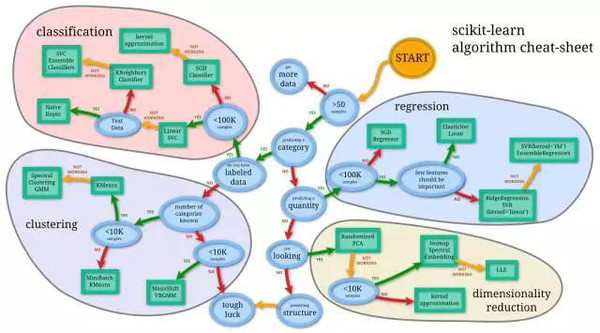3. 机器学习问题解决思路

3.1 数据与可视化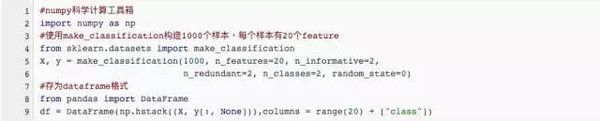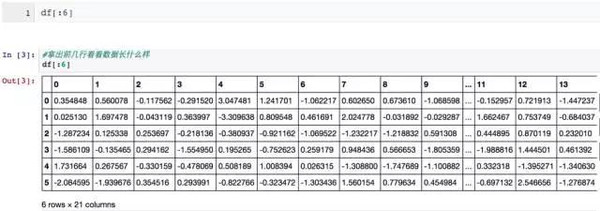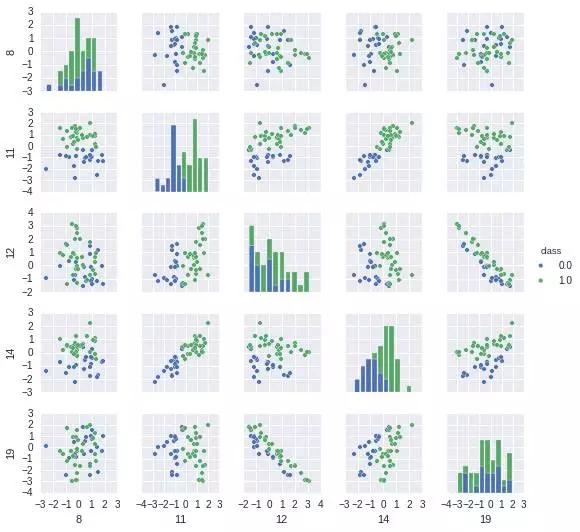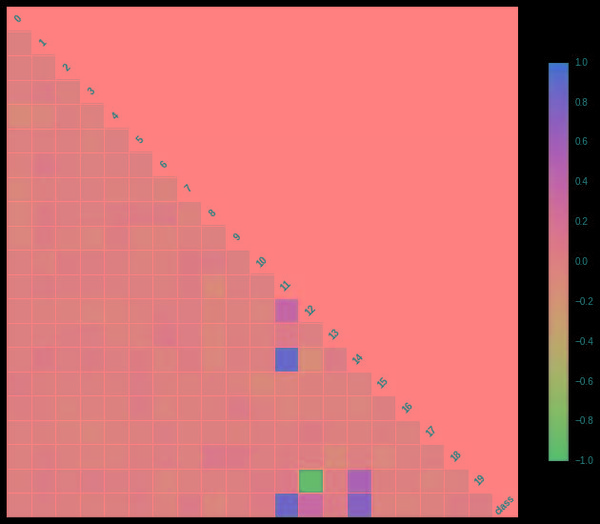3.2 机器学习算法选择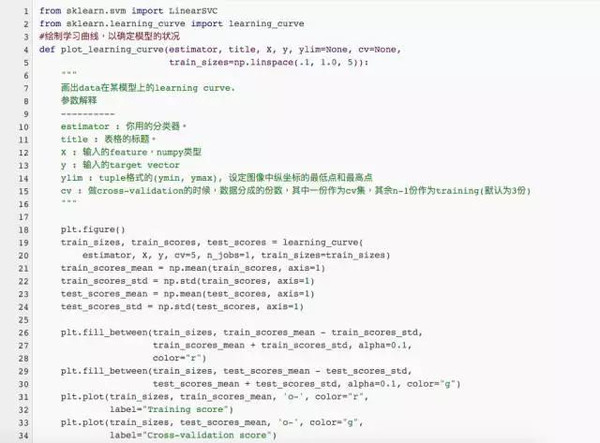3.2.1 过拟合的定位与解决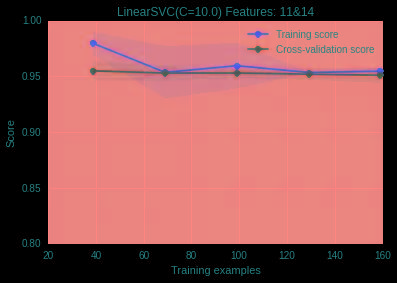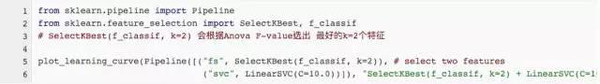(2)神经网络中减少神经网络的层数和每层的结点数 (c)SVM中增加RBF-kernel的bandwidth等方式来降低模型的复杂度。

l2正则化，它对于最后的特征权重的影响是，尽量打散权重到每个特征维度上，不让权重集中在某些维度上，出现权重特别高的特征。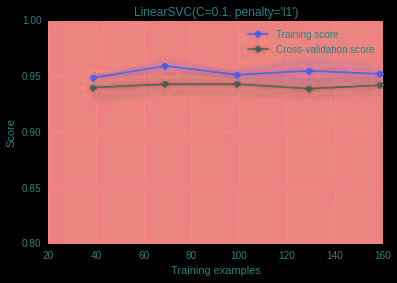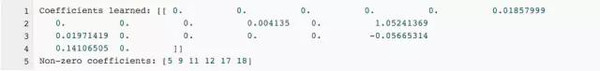3.2.2 欠拟合定位与解决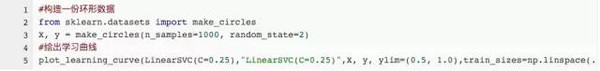3.3 关于大数据样本集和高维特征空间